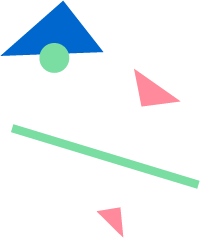# Left

Returns a substring beginning at the start of a given string.

## Usage

`Left(string, number)`

string (required) The string from which a left substring will be returned.

number (required) The desired length of the returned substring.

## Example

`Left("Dec. 12, 1950", 3)`
• Returns “Dec”
`Left("St. Louis", 20)`
• Returns “St. Louis”
• If number is greater than the length of string, the result is the original string.
`Left("San Francisco", -5)`
• Returns “San Fran”
• If you use a negative number, the result is the string with that number of characters removed from the end.